# Parallel displacement

A special case of a motion in which all points of the space are transferred in one and the same direction (along a line in that space) over one and the same distance. In other words, ifis the original and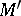is the shifted position of a point, then the vector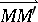is one and the same for all pairs of points corresponding to each other under the given transformation.

In the plane a parallel displacement may analytically be expressed in a rectangular coordinate system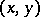by the formulas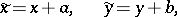(*)

where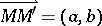.

The collection of all parallel displacements forms a group, which in a Euclidean space is a subgroup of the group of motions, and in an affine space is a subgroup of the group of affine transformations.

In the absolute plane a parallel displacement (cf. Absolute geometry) is the product of reflections in two parallel lines (cf. Reflection). Thus, in Euclidean geometry a parallel displacement is a translation, expressible in affine (or Cartesian) coordinates by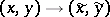(see (*)).